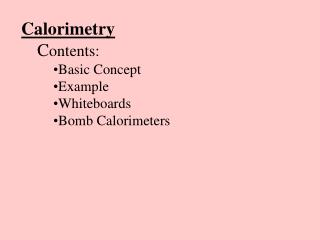Download PresentationCalorimetry C ontents: Basic Concept Example Whiteboards Bomb Calorimeters

# Calorimetry C ontents: Basic Concept Example Whiteboards Bomb Calorimeters - PowerPoint PPT PresentationDownload Presentation## Calorimetry C ontents: Basic Concept Example Whiteboards Bomb Calorimeters

- - - - - - - - - - - - - - - - - - - - - - - - - - - E N D - - - - - - - - - - - - - - - - - - - - - - - - - - -
##### Presentation Transcript

1. Calorimetry • Contents: • Basic Concept • Example • Whiteboards • Bomb Calorimeters

2. Calorimetry Drop a hot piece of material into water Heat lost by hot stuff = heat gained by cold stuff TOC

3. Calorimetry - Example 1 - finding c A .231 kg piece of unknown substance at 98 oC is dropped into .481 kg of water at 18 oC. The final temperature of the water is 32 oC. What is the specific heat of the substance? (neglect the calorimeter cup, and assume no heat is lost to the surroundings) (cwater = 4186 JoC-1kg-1) Heat lost = Heat gained m1c1T1 = m2c2T2 (.231)c(98-32) = (.481 kg)(4186)(32-18) c = 1848.9 = 1800 JoC-1kg-1 TOC

4. Calorimetry - find c or m 1 | 2 TOC

5. .112 kg of a mystery substance at 85.45 oC is dropped into .873 kg of water at 18.05 oC in an insulated Styrofoam container. The water and substance come to equilibrium at 23.12 oC. What is the c of the substance? (cwater = 4186 JoC-1kg-1) Heat lost = Heat gained m1c1T1 = m2c2T2 (.112)c(85.45-23.12) = (.873 kg)(4186)(23.12-18.05) c = 2650 JoC-1kg-1 W 2650 JoC-1kg-1

6. A chunk of Mippsalipsium at 68.1 oC is dropped into .625 kg of water at 21.1 oC in a .257 kg Aluminum calorimeter. The water, Aluminum, and Mippsalipsium come to equilibrium at 25.2 oC. What is the mass of the Mippsalipsium? (cwater = 4186 JoC-1kg-1, cAl = 900. JoC-1kg-1, cMi = 2174 JoC-1kg-1) Heat lost = Heat gained m1c1T1 = m2c2T2 +m3c3T3 m(2174)(68.1-25.2) = (.625)(4186)(25.2-21.1) + (.257)(900.)(25.2-21.1) m = .125 kg W .125 kg

7. Calorimetry - Example 2 - Finding T A .250 kg piece of iron at 95.0 oC is dropped into .512 kg of water at 18.0 oC. What is the final equilibrium temperature? (neglect the calorimeter cup, and assume no heat is lost to the surroundings) (cwater = 4186 JoC-1kg-1,cFe = 450. JoC-1kg-1) Heat lost = Heat gained m1c1T1 = m2c2T2 (.250)(450)(95-T) = (.512 kg)(4186)(T-18) (explain how to set up equation) (show how to do math) T = 21.845 oC = 21.8 oC TOC

8. Calorimetry - find T 1 | 2 TOC

9. 52 grams of glass at 91.1 oC is dropped into 154 g of water at 25.1 oC in an insulated Styrofoam container. What will be the final equilibrium temperature if no heat is lost to the surroundings? (cwater = 4186 JoC-1kg-1, cglass = 840 JoC-1kg-1) Heat lost = Heat gained m1c1T1 = m2c2T2 (.052)(840)(91.1-T) = (.154 kg)(4186)(T-25.1) T = 29 oC W 29 oC

10. 127 grams of copper at 99.5 oC is dropped into 325 g of water at 23.6 oC in a 562 g glass beaker. What will be the final equilibrium temperature if no heat is lost to the surroundings? (cwater = 4186 JoC-1kg-1, cglass = 840 JoC-1kg-1, cCu = 390 JoC-1kg-1) Heat lost = Heat gained m1c1T1 = m2c2T2 +m3c3T3 (.127)(390)(99.5-T) = (.325)(4186)(T-23.6) + (.562)(840)(T-23.6) T = 25.6 oC W 25.6 oC

11. Bomb Calorimeters The Bomb with water jacket The Bomb Candy bar/O2/electric ignition/temperature rise TOC

12. Bomb Calorimetry 1 TOC

13. 3.215 g of a candy bar is placed in a 3.687 kg bomb calorimeter, surrounded by 2.150 kg of water. The entire apparatus is happily at 22.25 oC before detonation, and the temperature rises to 33.12 oC after. Ignore the heat retained by the combustion products. A) What is the energy released to the calorimeter and water in kilojoules? (cwater = 4186 JoC-1kg-1, cAl = 900. JoC-1kg-1) B) What is the caloric content in Kilocalories (C) if the entire candy bar has a mass of 35 grams. 1 cal = 4.18 J Heat released = m1c1T1 + m2c2T2 Heat = (3.687)(900.)(33.12-22.25) + (2.150)(4186)(33.12-22.25) Heat = 136856.4523 J = 137 kJ Total energy = 133.76*35/4.18/3.215 = 356 kc = 356 C W 134 kJ, 348 C